# MongoDB – Less than equals to Operator \$lte

• Last Updated : 27 Mar, 2020

MongoDB provides different types of comparison operators and less than equals to operator( \$lte ) is one of them. This operator is used to select those documents where the value of the field is less than equals to(<=) the given value. You can use this operator in methods like `find()`, `update()`, etc. according to your requirements.

Syntax:

`{field: {\$lte: value}}`

In the following examples, we are working with:

Database: GeeksforGeeks
Collection: employee
Document: four documents that contain the details of the employees in the form of field-value pairs.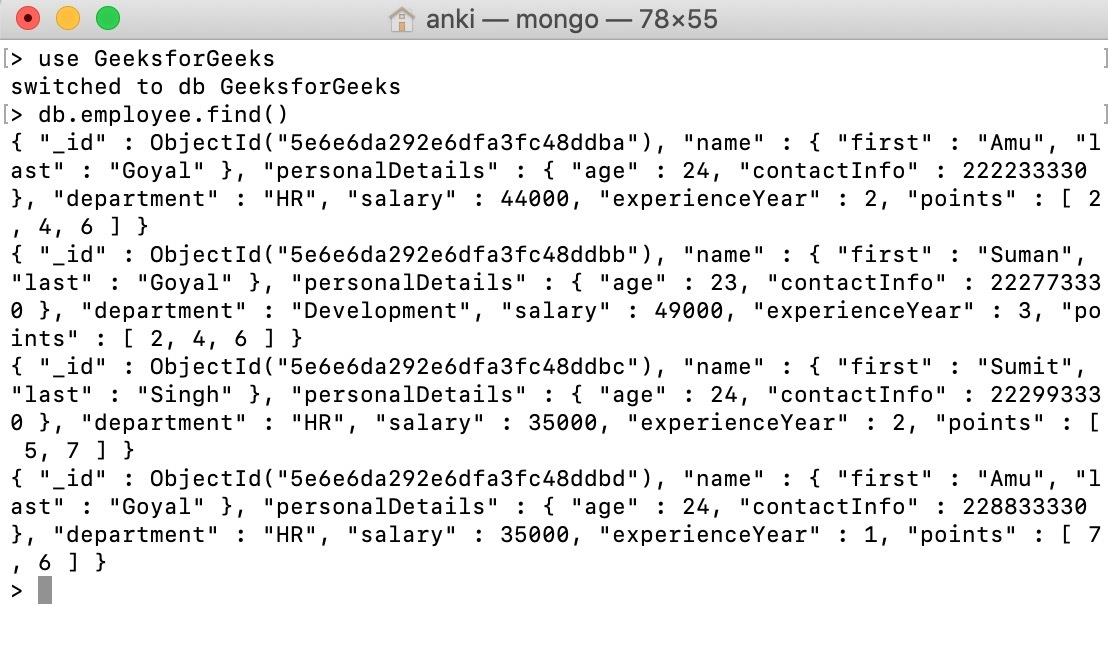Example #1:
In this example, we are selecting those documents where the value of the salary field is less than equals to 35000.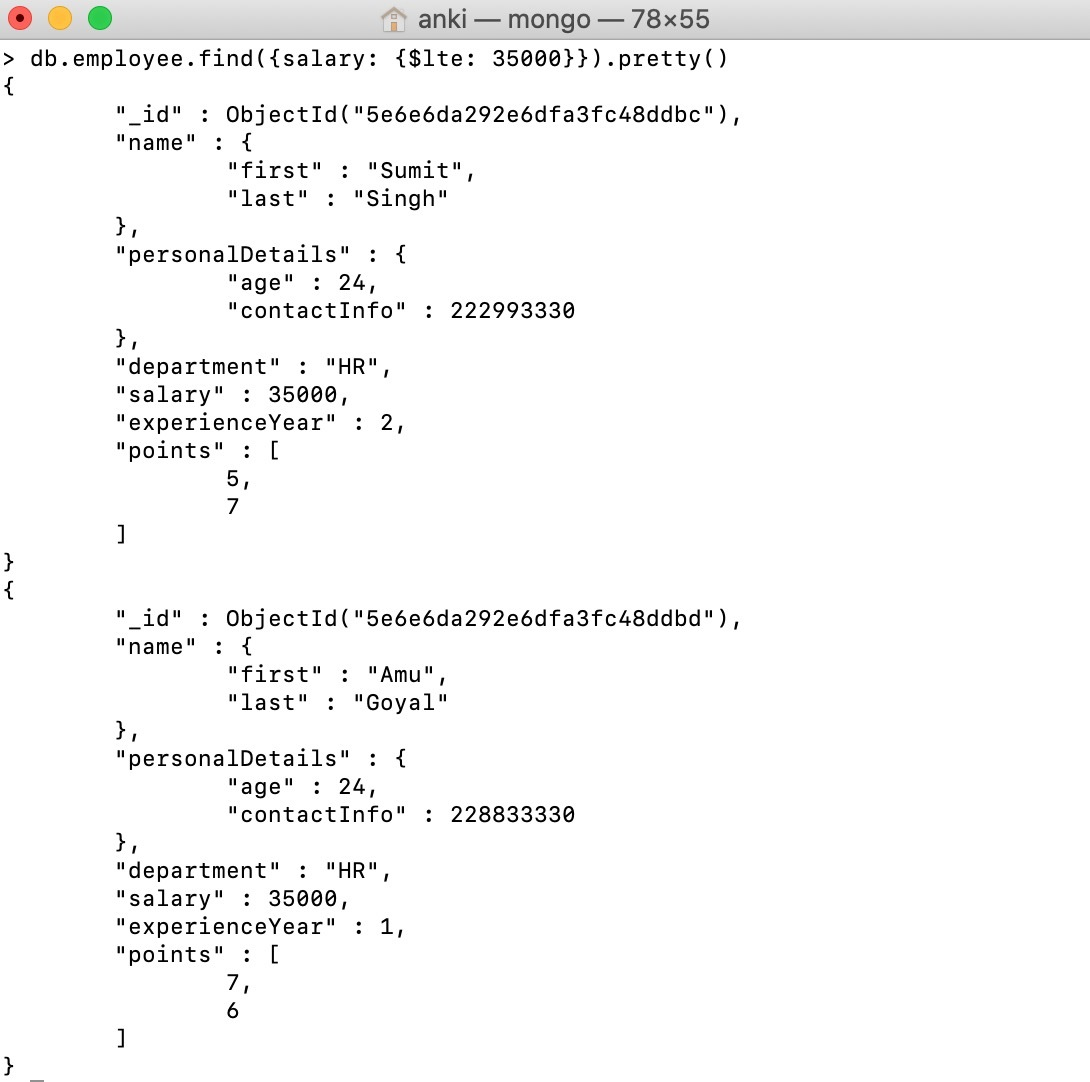Example #2:
In this example, we are selecting only those documents where the age of the employee is less than equals to 24. Or in other words, in this example, we are specifying conditions on the field in the embedded document using dot notation.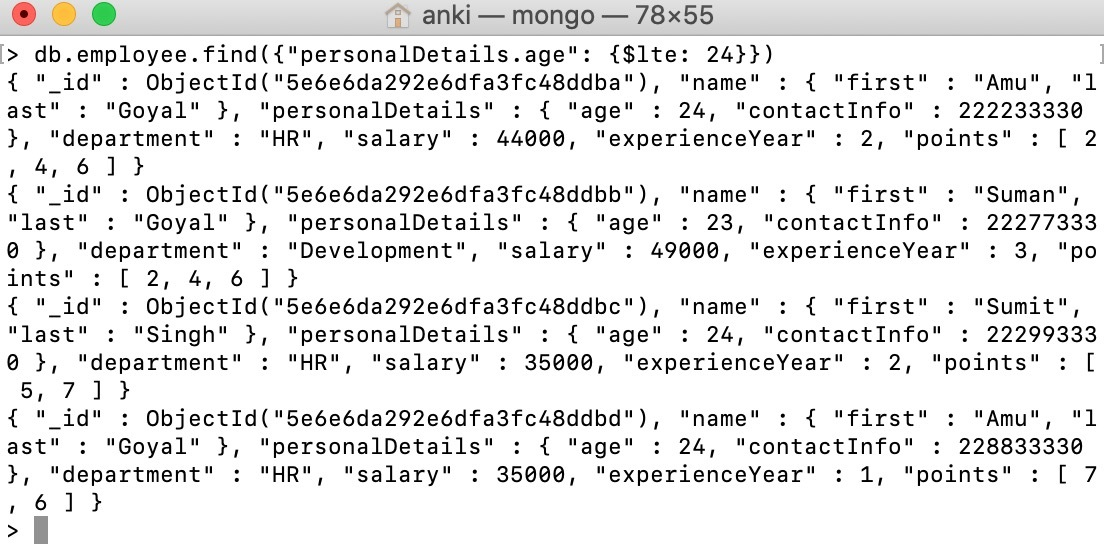Example #3:
In this example, we are selecting only those documents where the points array is less than equals to the specified array.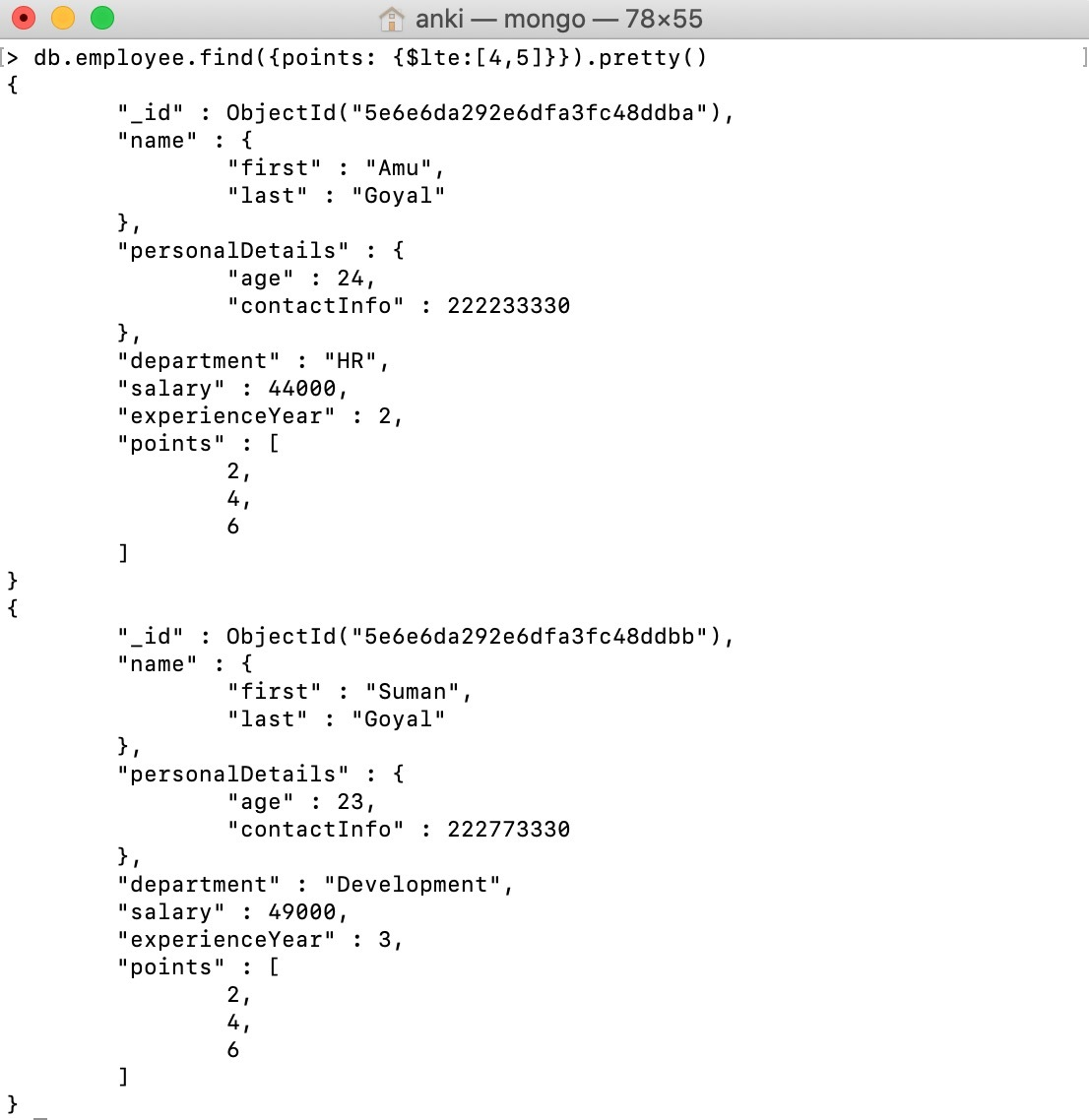Example #4:
In this example, we are updating the salary of those employees whose experience year is less than equals to 2 years. Or in other words, set the value of the salary field to 32000 of those documents whose experienceYear field value is less than equals to 2.

Note: The `update()` method by default update only one document at a time. If you want to update multiple documents, then set the value of its multi parameter to true. So, in this example, the update() method updated the first document that matches the given condition as shown in the below image.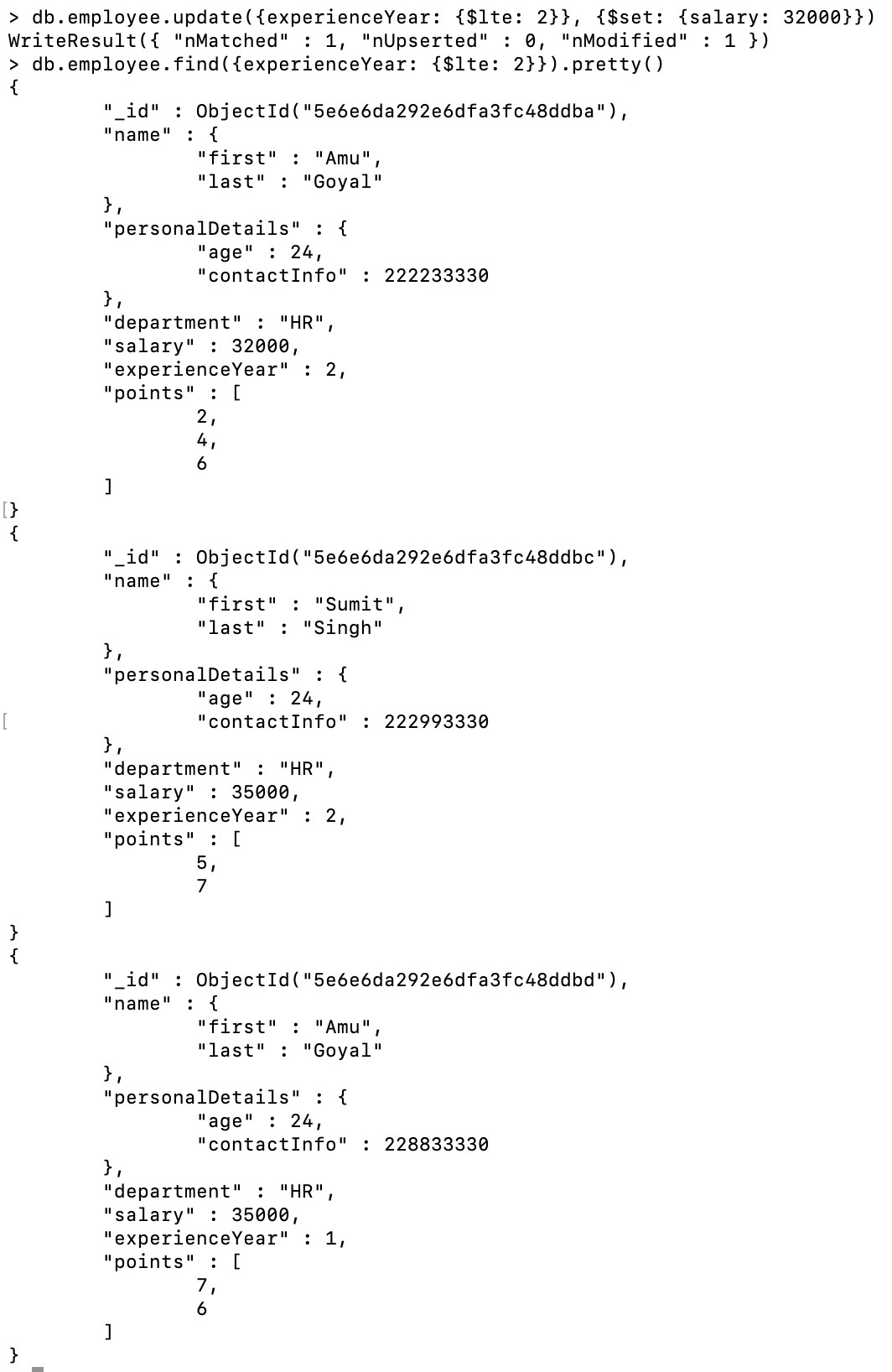My Personal Notes arrow_drop_up